Math resources Geometry

# Geometry

Here you will find all the geometry resources you need. Like all our math topic guides, we’ve included step-by-step guides, detailed examples and practice questions!

Filter by:

Area

Lines

2D shapes

Angles

Perimeter

Volume

3D shapes

Surface area

Triangles

Show all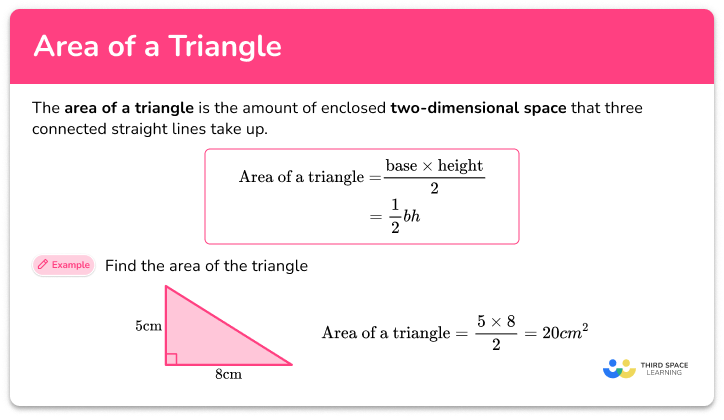Area of a triangle

Area of a triangle step-by-step topic guide, detailed examples, practice questions, teaching tips and FAQs.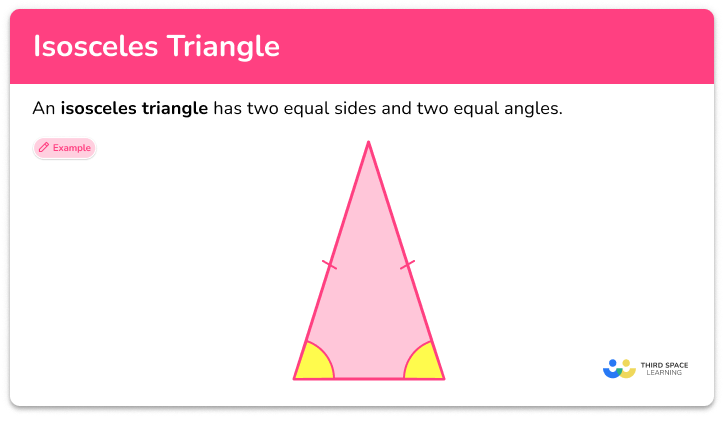Isosceles triangle

Isosceles triangle step-by-step topic guide, detailed examples, practice questions, teaching tips and FAQs.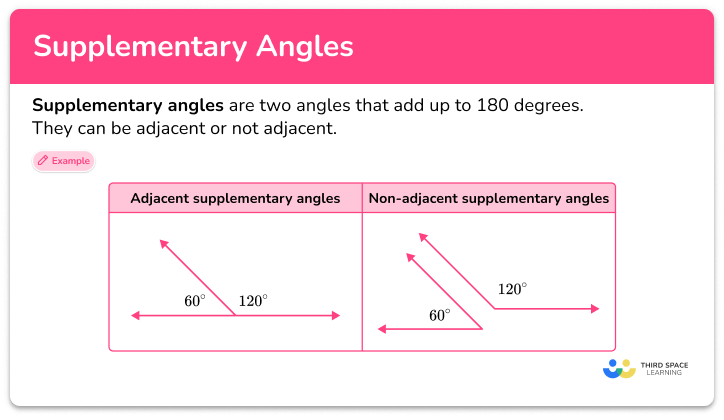Supplementary angles

Supplementary angles step-by-step topic guide, detailed examples, practice questions, teaching tips and FAQs.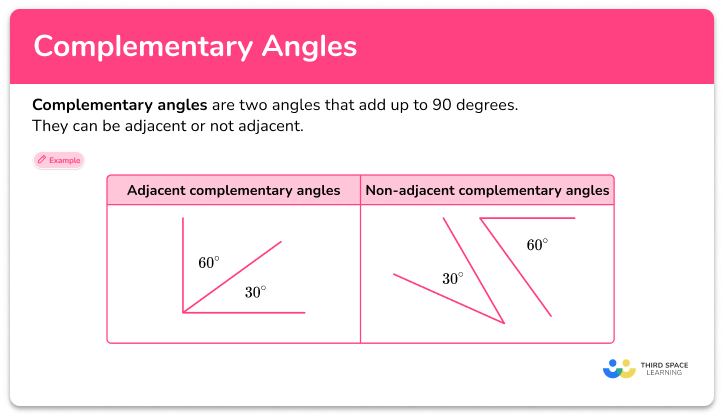Complementary angles

Complementary angles step-by-step topic guide, detailed examples, practice questions, teaching tips and FAQs.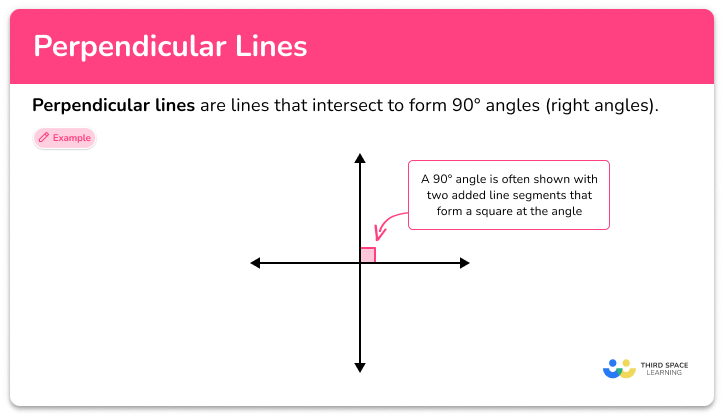Perpendicular lines

Perpendicular lines step-by-step topic guide, detailed examples, practice questions, teaching tips and FAQs.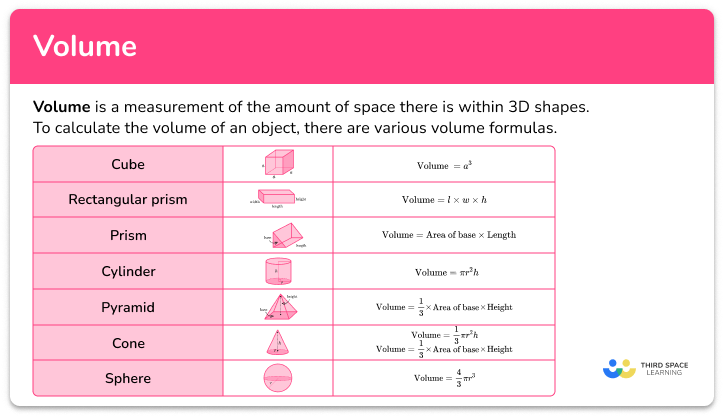Volume

Volume step-by-step topic guide, detailed examples, practice questions, teaching tips and FAQs.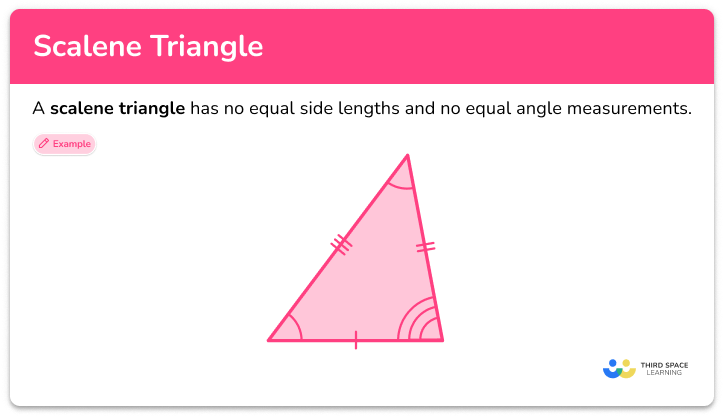Scalene triangle

Scalene triangles step-by-step topic guide, detailed examples, practice questions, teaching tips and FAQs.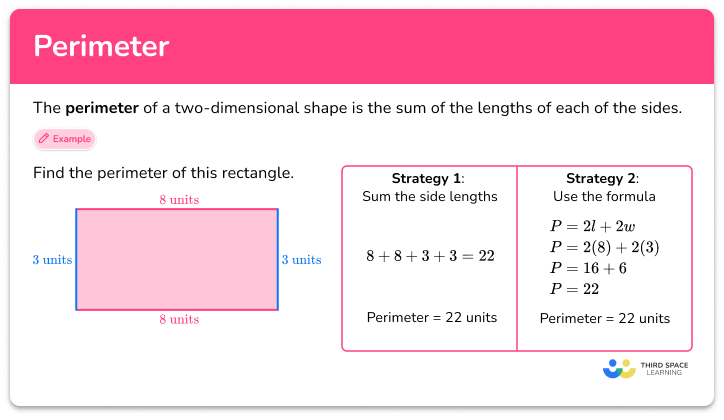Perimeter

Perimeter step-by-step topic guide, detailed examples, practice questions, teaching tips and FAQs.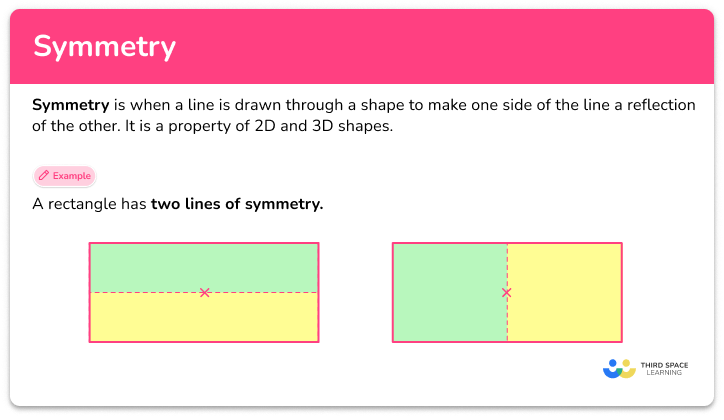Symmetry

Symmetry step-by-step topic guide, detailed examples, practice questions, teaching tips and FAQs.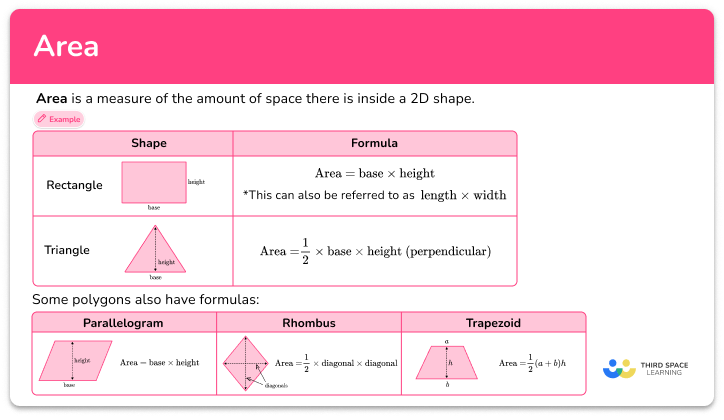Area

Area step-by-step topic guide, detailed examples, practice questions, teaching tips and FAQs.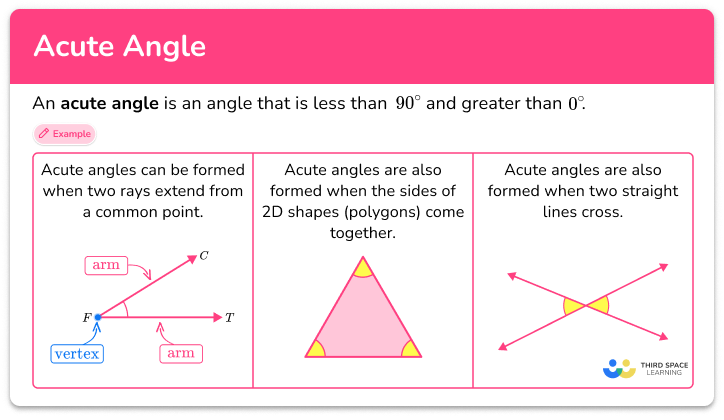Acute angle

Acute angle step-by-step topic guide, detailed examples, practice questions, teaching tips and FAQs.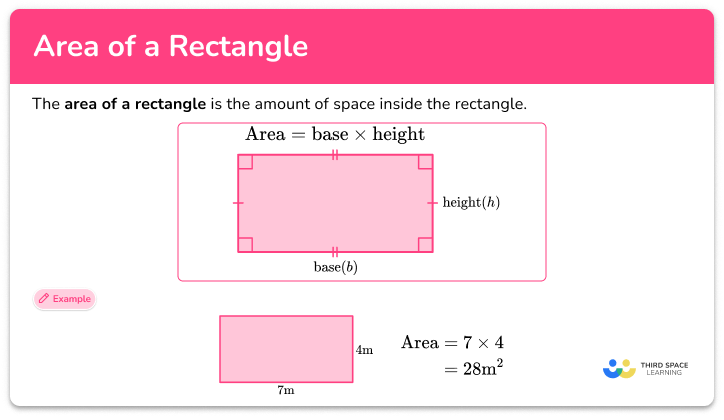Area of a rectangle

Area of a rectangle step-by-step topic guide, detailed examples, practice questions, teaching tips and FAQs.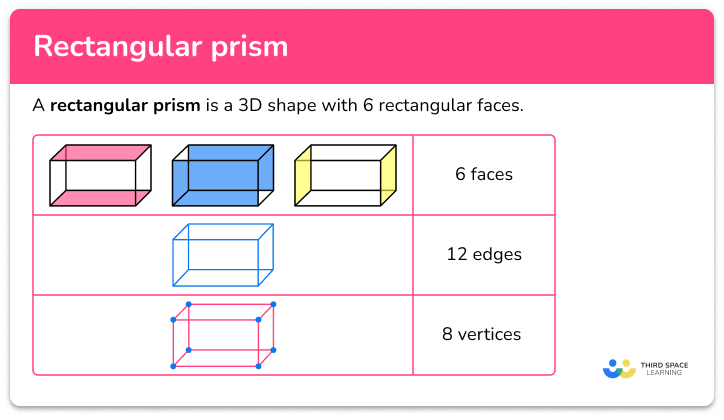Rectangular prism

Rectangular prism step-by-step topic guide, detailed examples, practice questions, teaching tips and FAQs.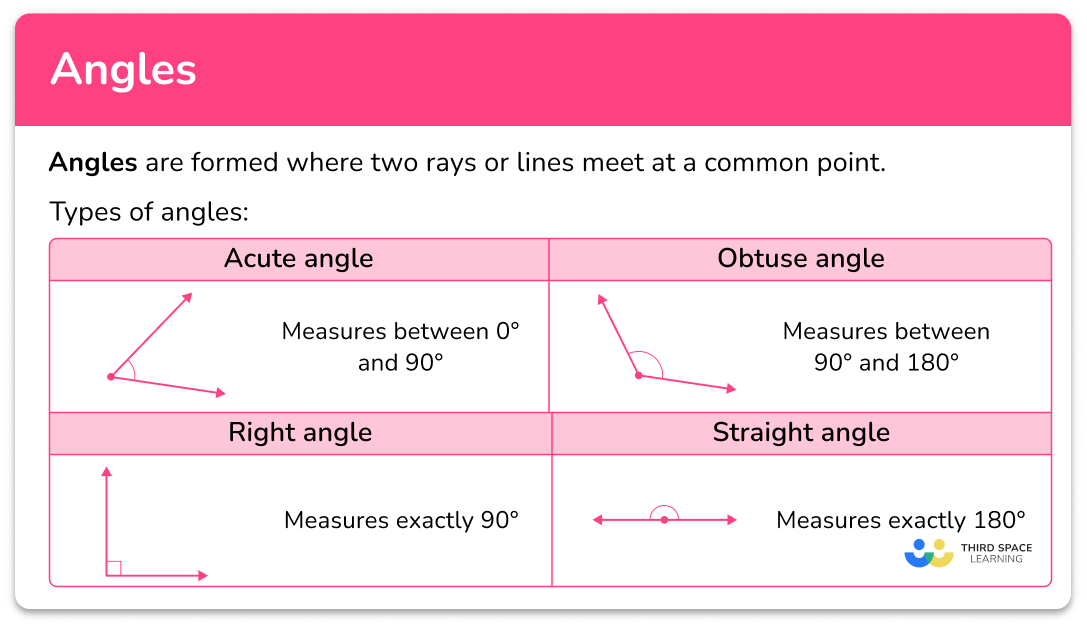Angles

Angles step-by-step topic guide, detailed examples, practice questions, teaching tips and FAQs.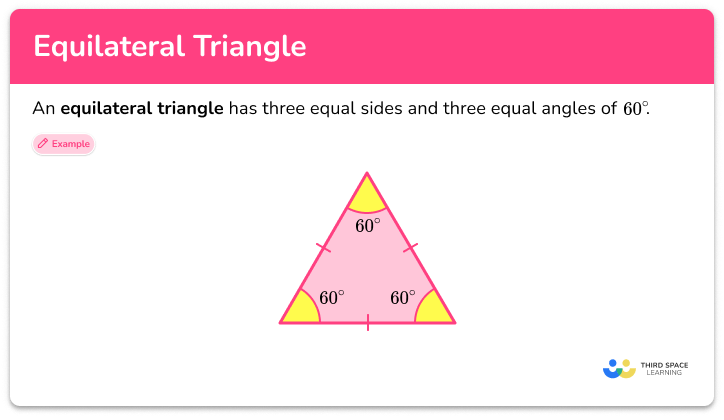Equilateral triangle

Equilateral triangle step-by-step topic guide, detailed examples, practice questions, teaching tips and FAQs.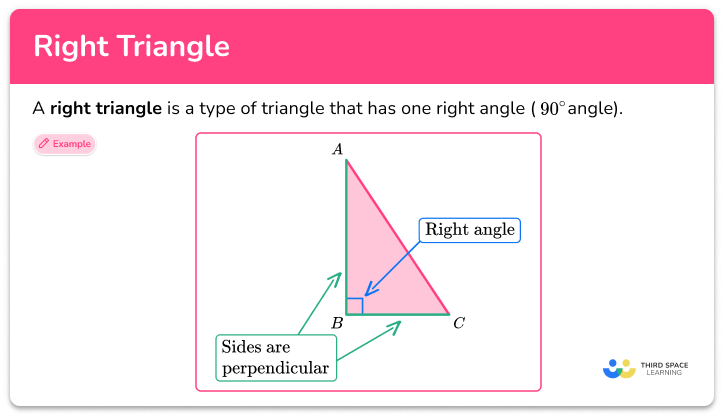Right triangle

Right triangle step-by-step topic guide, detailed examples, practice questions, teaching tips and FAQs.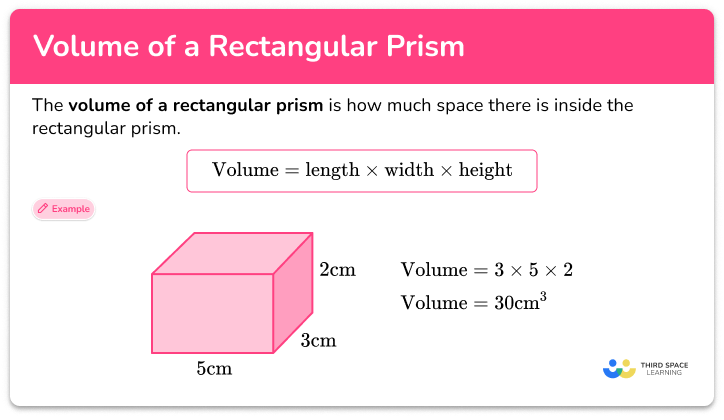Volume of a rectangular prism

Volume of a rectangular prism step-by-step topic guide, detailed examples, practice questions, teaching tips and FAQs.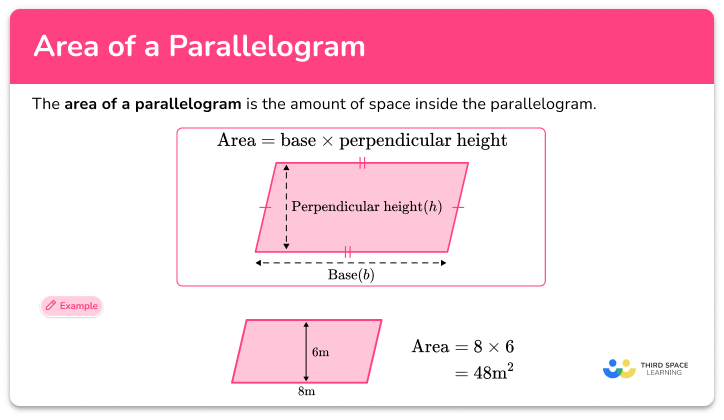Area of a parallelogram

Area of a parallelogram step-by-step topic guide, detailed examples, practice questions, teaching tips and FAQs.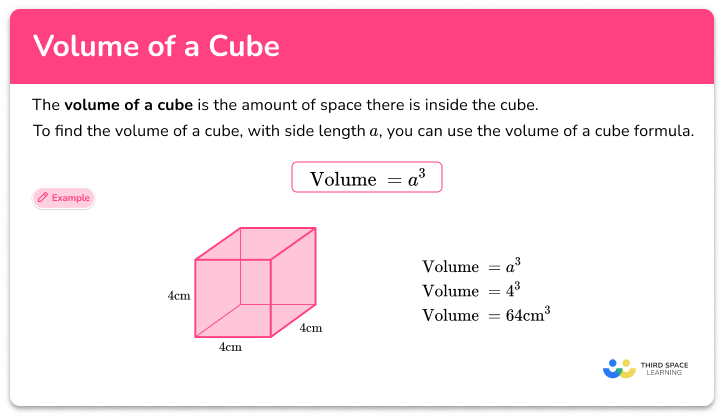Volume of a cube

Volume of a cube step-by-step topic guide, detailed examples, practice questions, teaching tips and FAQs.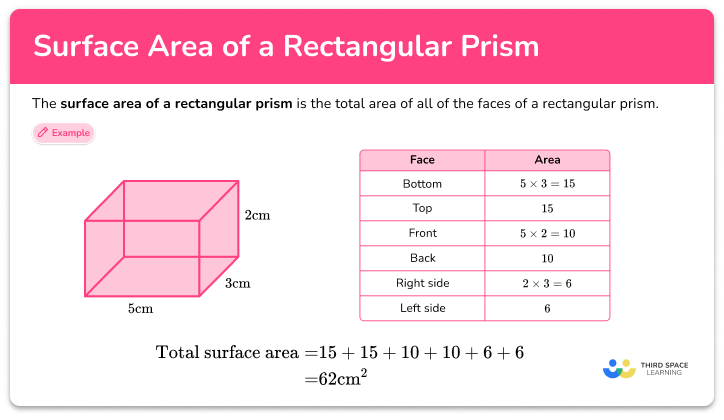Surface area of a rectangular prism

Surface area of a rectangular prism step-by-step topic guide, detailed examples, practice questions, teaching tips and FAQs.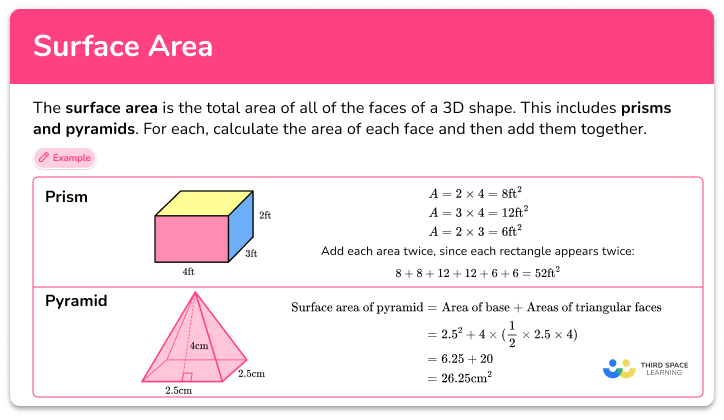Surface area

Surface area step-by-step topic guide, detailed examples, practice questions, teaching tips and FAQs.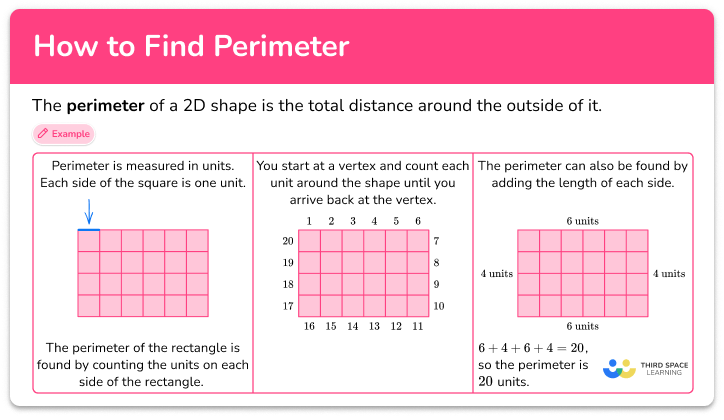How to find perimeter

How to find perimeter step-by-step topic guide, detailed examples, practice questions, teaching tips and FAQs.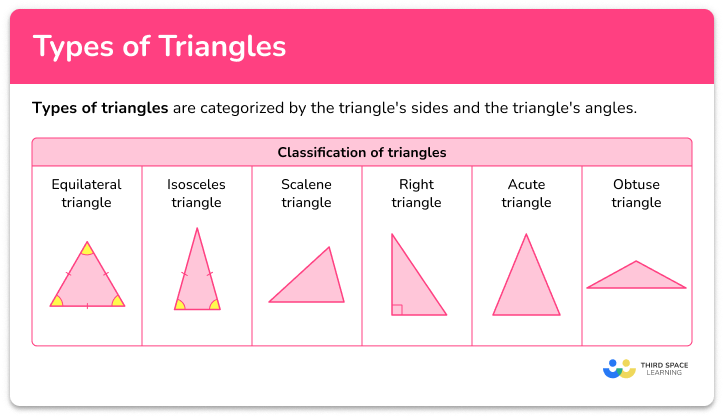Types of triangles

Types of triangles step-by-step topic guide, detailed examples, practice questions, teaching tips and FAQs.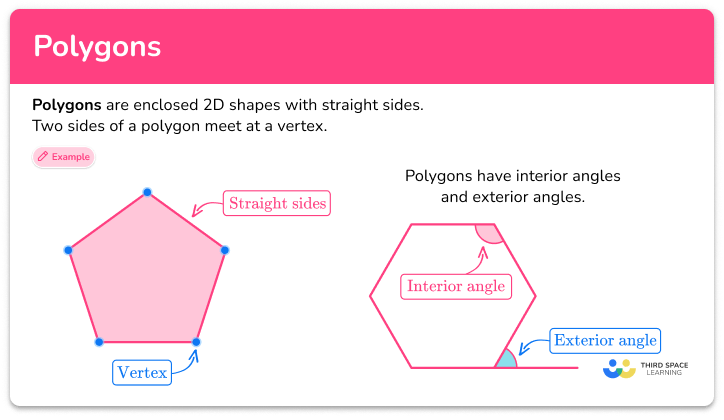Polygons

Polygons step-by-step topic guide, detailed examples, practice questions, teaching tips and FAQs.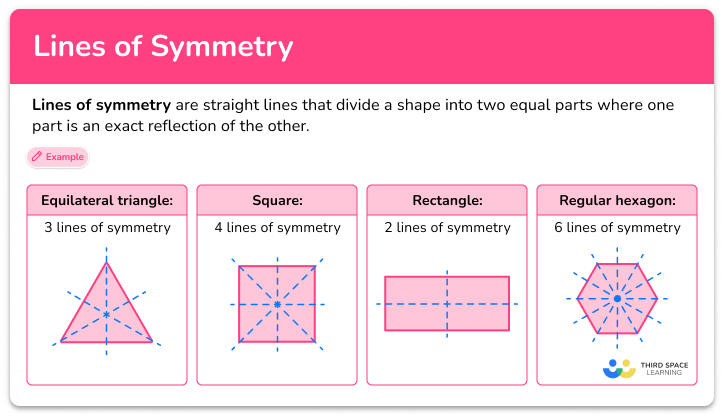Lines of symmetry

Lines of symmetry step-by-step topic guide, detailed examples, practice questions, teaching tips and FAQs.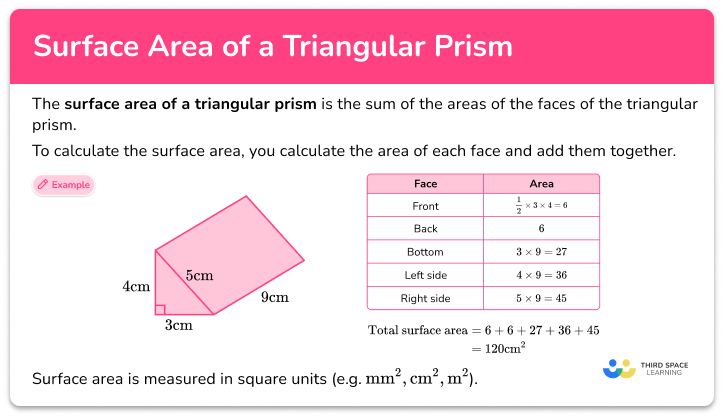Surface area of a triangular prism

Surface area of a triangular prism step-by-step topic guide, detailed examples, practice questions, teaching tips and FAQs.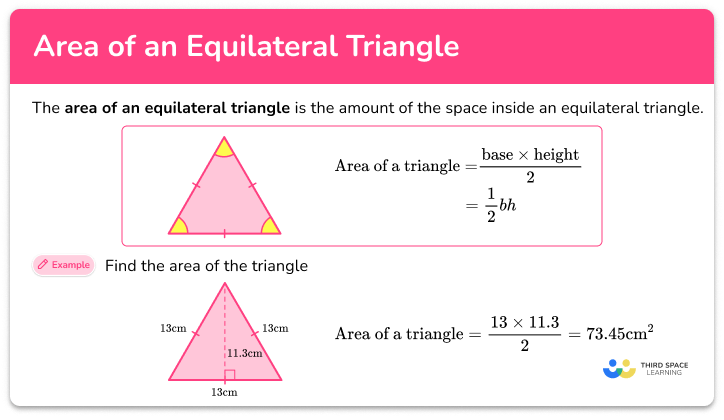Area of equilateral triangle

Area of equilateral triangle step-by-step topic guide, detailed examples, practice questions, teaching tips and FAQs.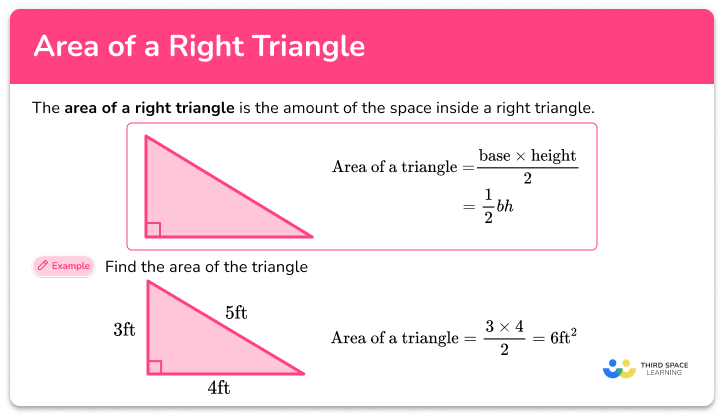Area of a right triangle

Area of a right triangle step-by-step topic guide, detailed examples, practice questions, teaching tips and FAQs.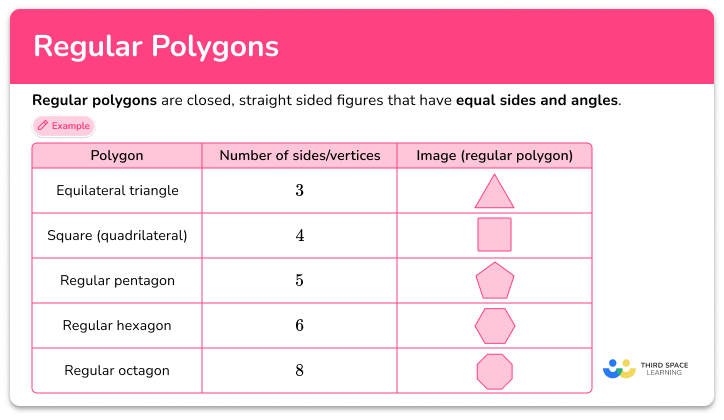Regular polygon

Regular polygon step-by-step topic guide, detailed examples, practice questions, teaching tips and FAQs.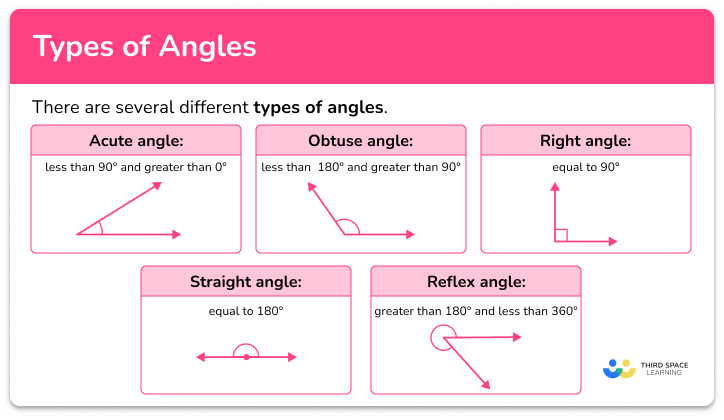Types of angles

Types of angles step-by-step topic guide, detailed examples, practice questions, teaching tips and FAQs.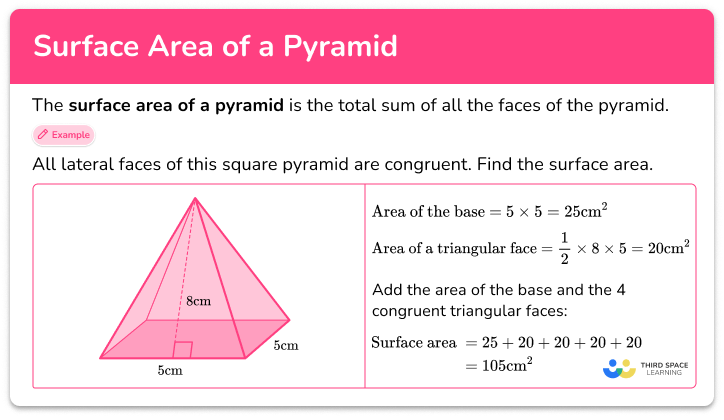Surface area of a pyramid

Surface area of a pyramid step-by-step topic guide, detailed examples, practice questions, teaching tips and FAQs.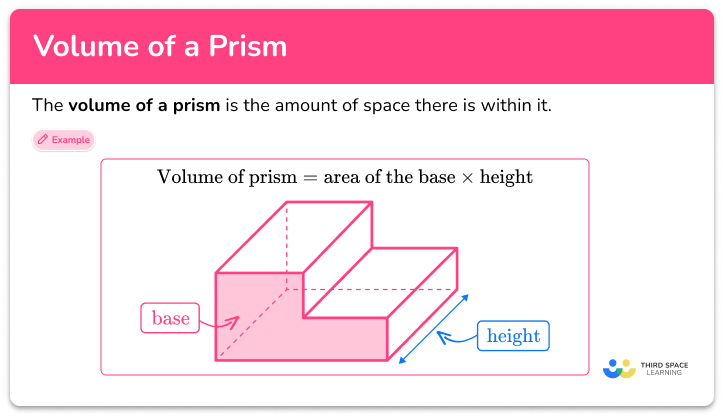Volume of a prism

Volume of a prism step-by-step topic guide, detailed examples, practice questions, teaching tips and FAQs.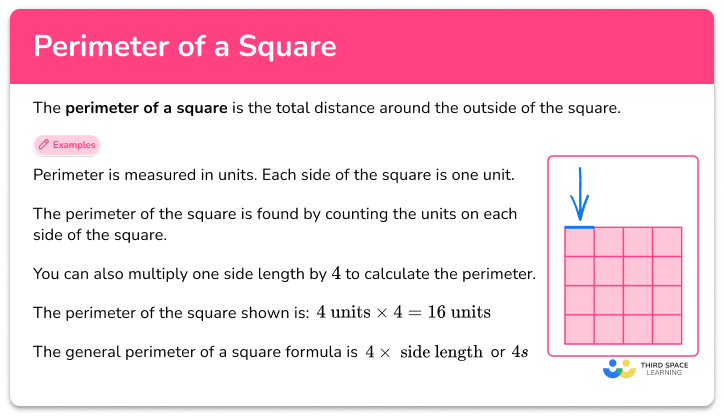Perimeter of a square

Perimeter of a square step-by-step topic guide, detailed examples, practice questions, teaching tips and FAQs.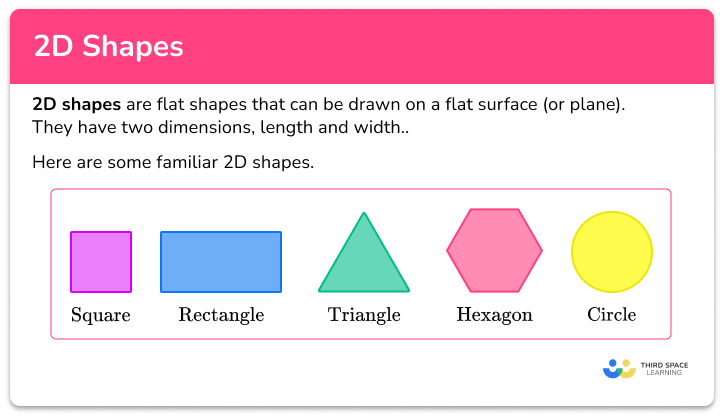2D shapes

2D shapes step-by-step topic guide, detailed examples, practice questions, teaching tips and FAQs.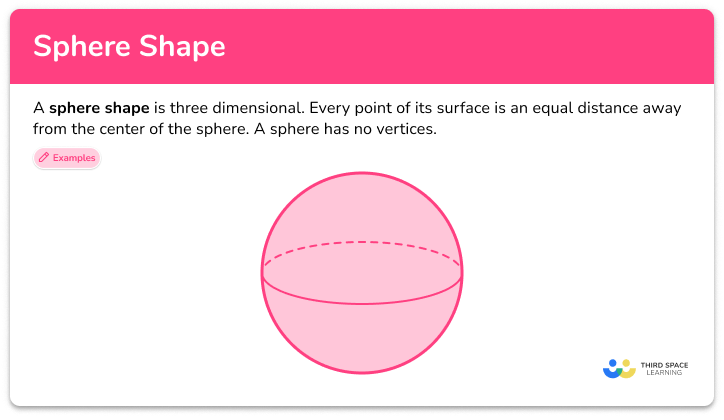Sphere shape

Sphere shape step-by-step topic guide, detailed examples, practice questions, teaching tips and FAQs.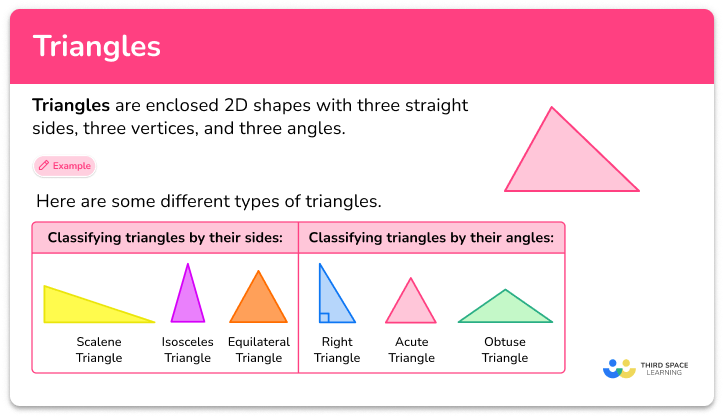Triangles

Triangles step-by-step topic guide, detailed examples, practice questions, teaching tips and FAQs.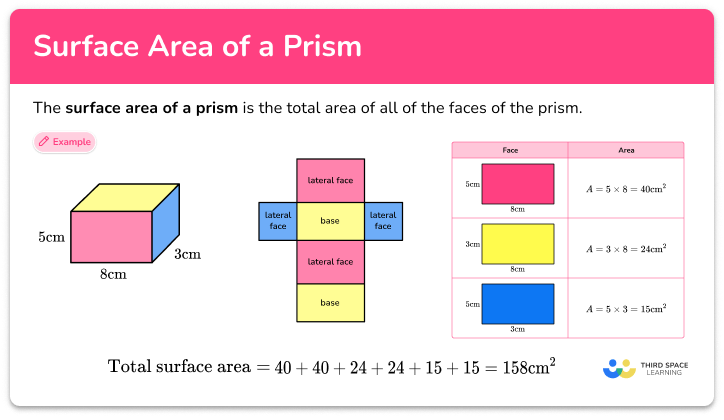Surface area of a prism

Surface area of a prism step-by-step topic guide, detailed examples, practice questions, teaching tips and FAQs.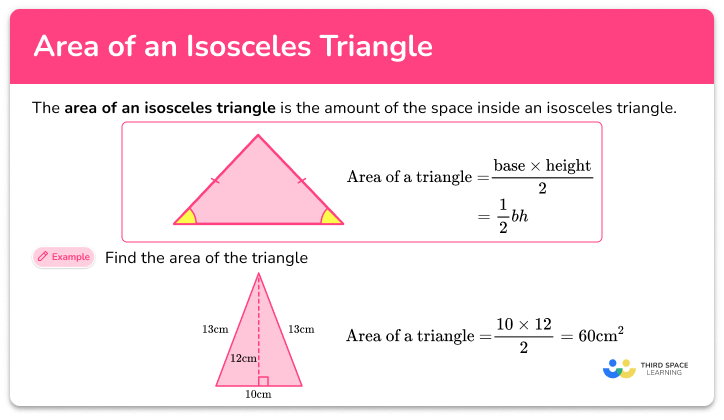Area of isosceles triangle

Area of isosceles triangle step-by-step topic guide, detailed examples, practice questions, teaching tips and FAQs.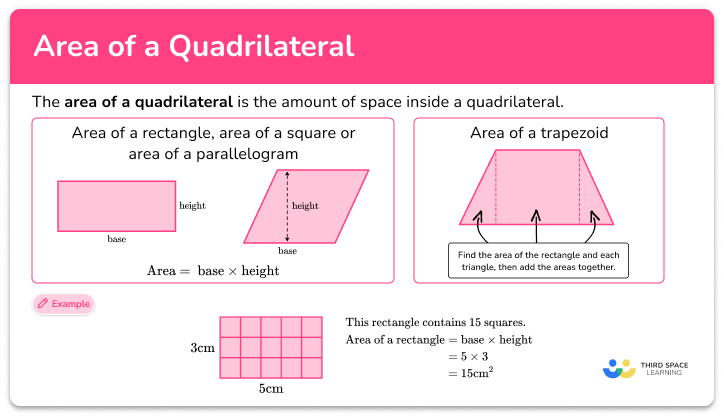Area of a quadrilateral step-by-step topic guide, detailed examples, practice questions, teaching tips and FAQs.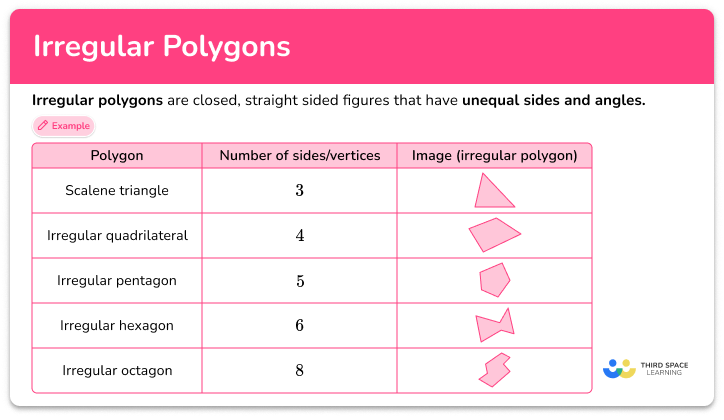Irregular polygon

Irregular polygon step-by-step topic guide, detailed examples, practice questions, teaching tips and FAQs.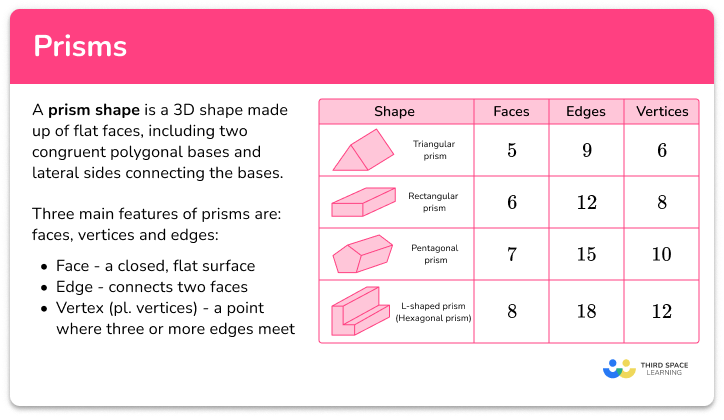Prism shape

Prism shape step-by-step topic guide, detailed examples, practice questions, teaching tips and FAQs.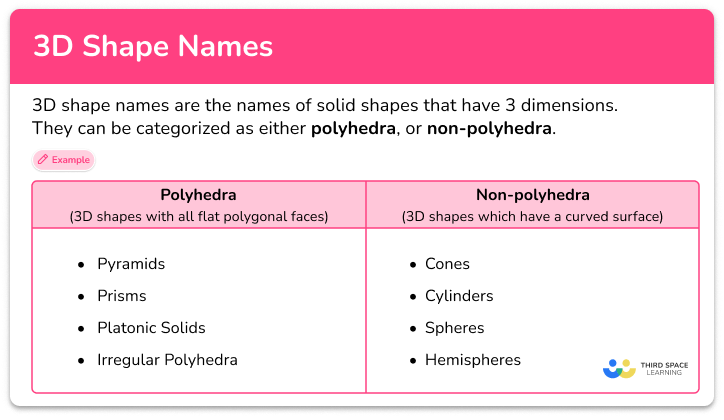3D shape names

3D shape names step-by-step topic guide, detailed examples, practice questions, teaching tips and FAQs.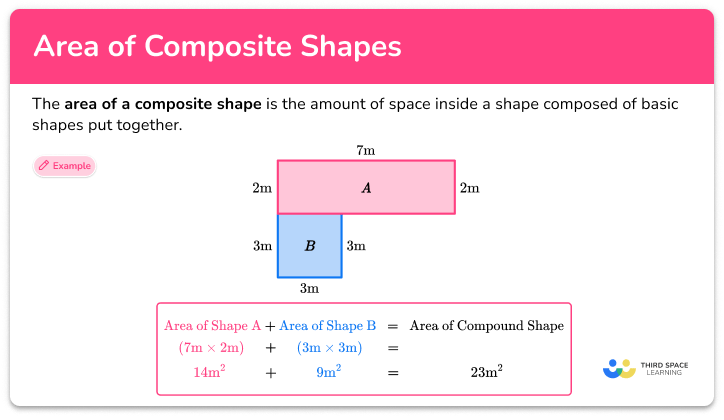Area of composite shapes

Area of composite shapes step-by-step topic guide, detailed examples, practice questions, teaching tips and FAQs.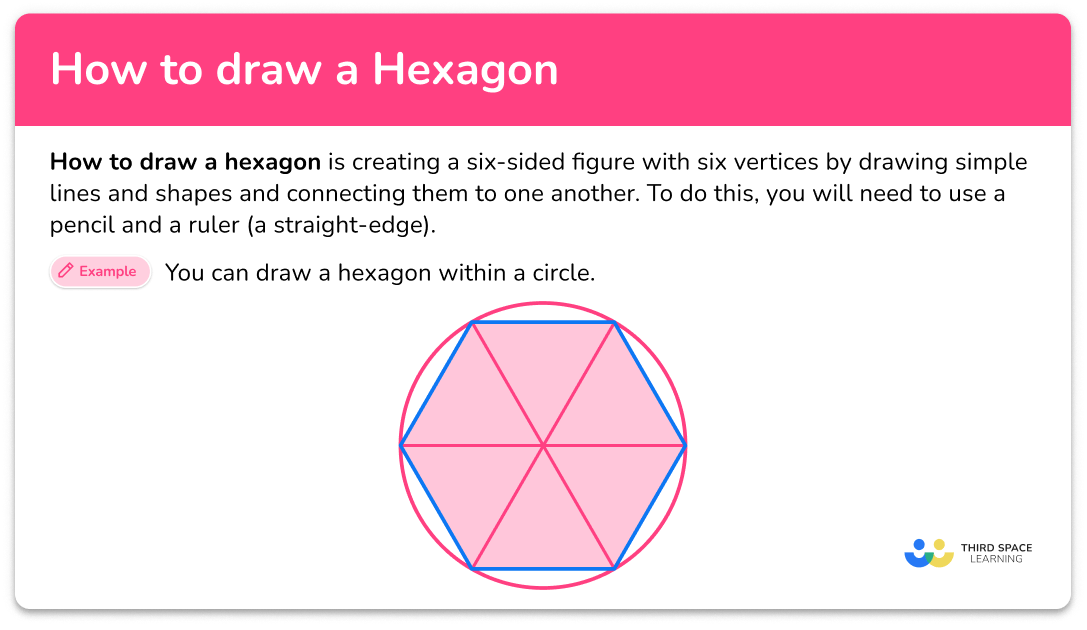How to draw a hexagon

How to draw a hexagon step-by-step topic guide, detailed examples, practice questions, teaching tips and FAQs.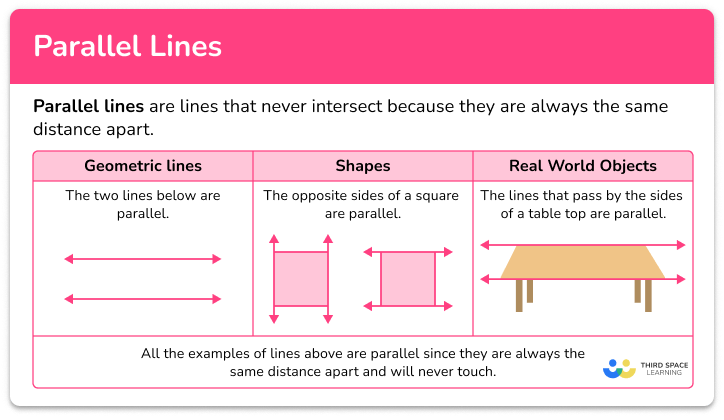Parallel lines

Parallel lines step-by-step topic guide, detailed examples, practice questions, teaching tips and FAQs.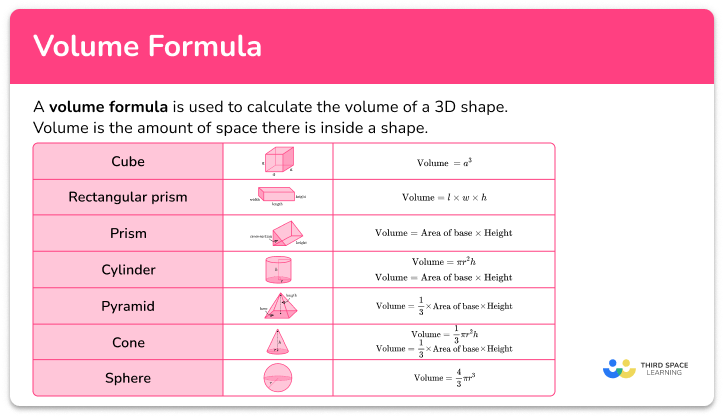Volume formula

Volume formula step-by-step topic guide, detailed examples, practice questions, teaching tips and FAQs.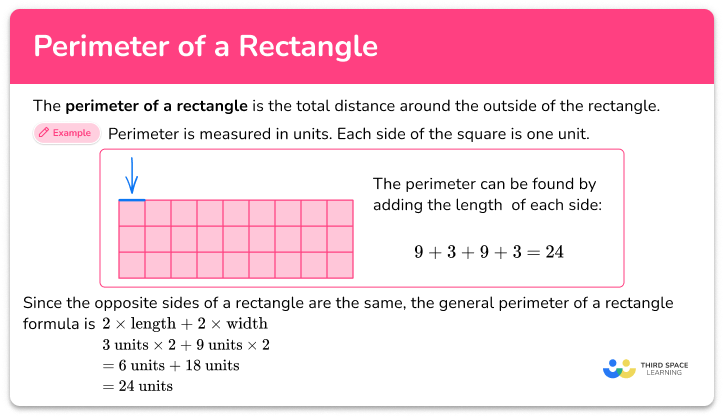Perimeter of a rectangle

Perimeter of a rectangle step-by-step topic guide, detailed examples, practice questions, teaching tips and FAQs.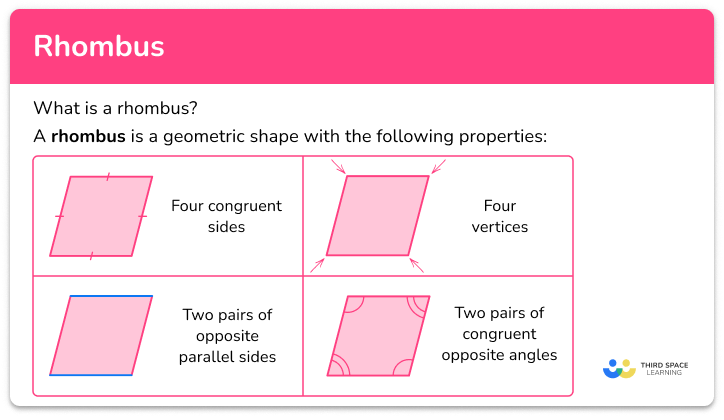Rhombus

Rhombus step-by-step topic guide, detailed examples, practice questions, teaching tips and FAQs.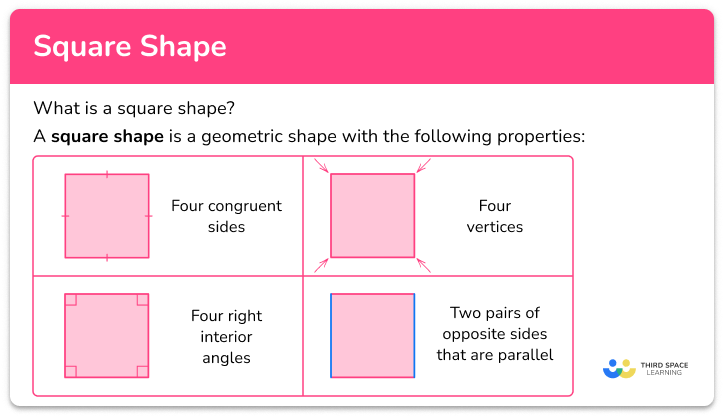Square shape

Square shape step-by-step topic guide, detailed examples, practice questions, teaching tips and FAQs.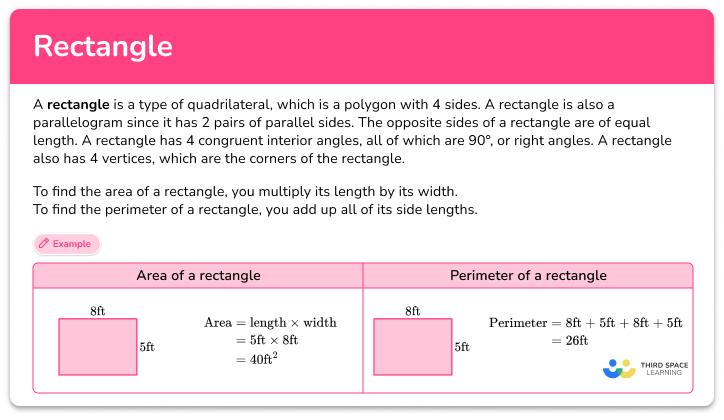Rectangle

Rectangle step-by-step topic guide, detailed examples, practice questions, teaching tips and FAQs.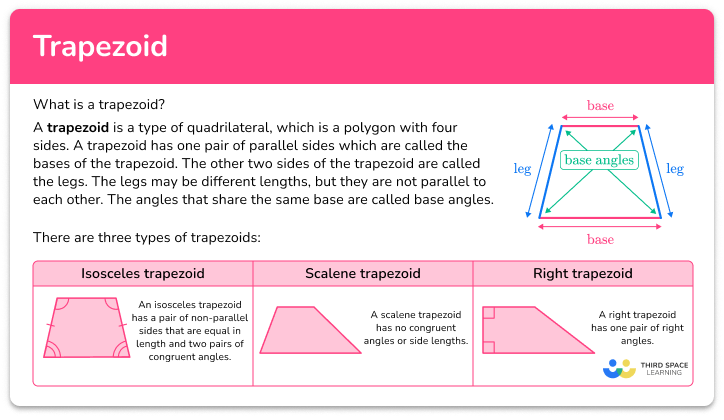Trapezoid

Trapezoid step-by-step topic guide, detailed examples, practice questions, teaching tips and FAQs.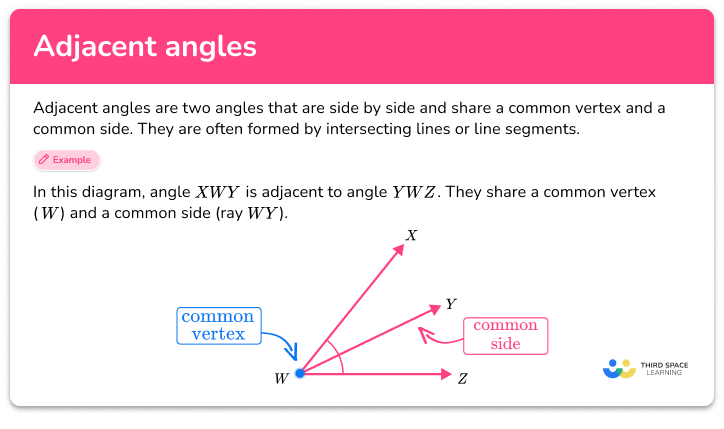Adjacent angles step-by-step topic guide, detailed examples, practice questions, teaching tips and FAQs.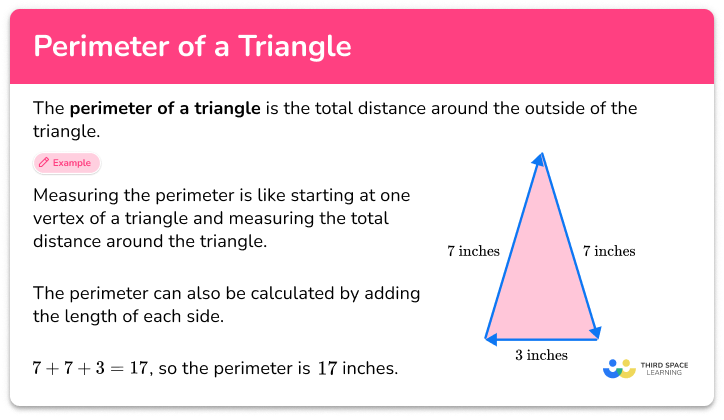Perimeter of a triangle

Perimeter of a triangle step-by-step topic guide, detailed examples, practice questions, teaching tips and FAQs.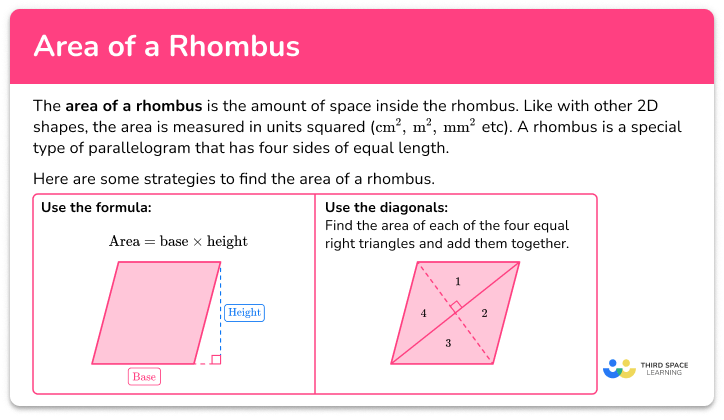Area of a rhombus

Area of a rhombus step-by-step topic guide, detailed examples, practice questions, teaching tips and FAQs.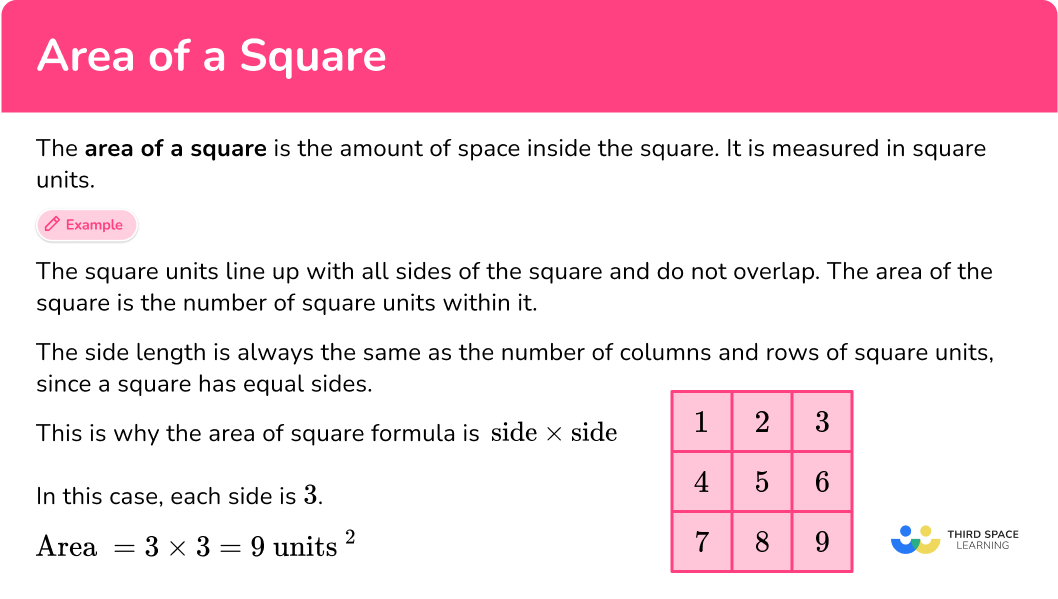Area of a square

Area of a square step-by-step topic guide, detailed examples, practice questions, teaching tips and FAQs.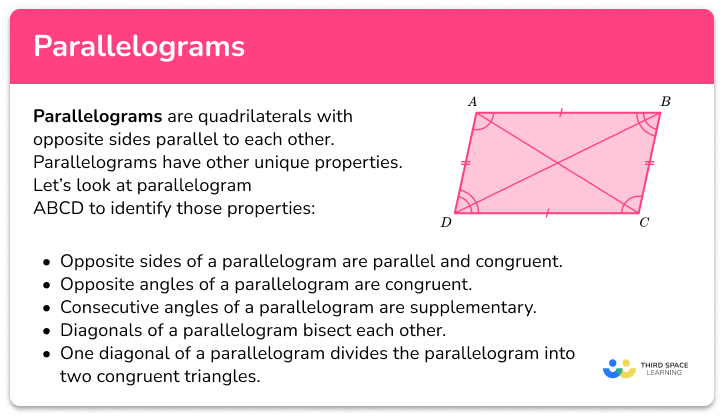Parallelogram

Parallelogram step-by-step topic guide, detailed examples, practice questions, teaching tips and FAQs.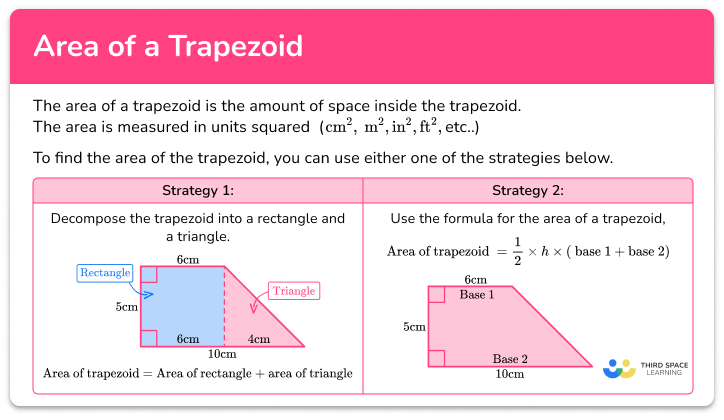Area of a trapezoid

Area of a trapezoid step-by-step topic guide, detailed examples, practice questions, teaching tips and FAQs.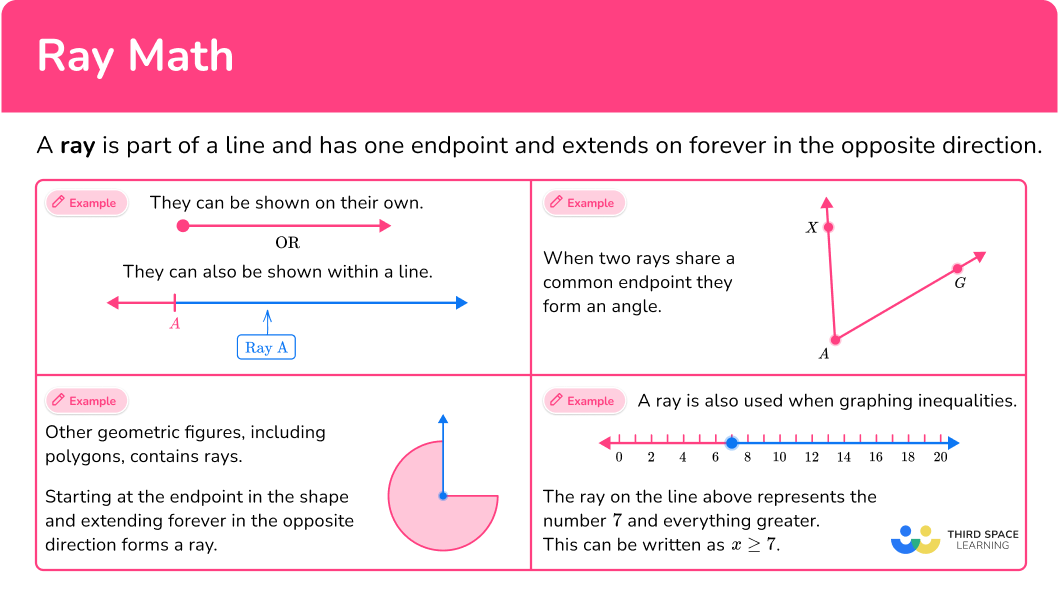Ray math

Ray math step-by-step topic guide, detailed examples, practice questions, teaching tips and FAQs.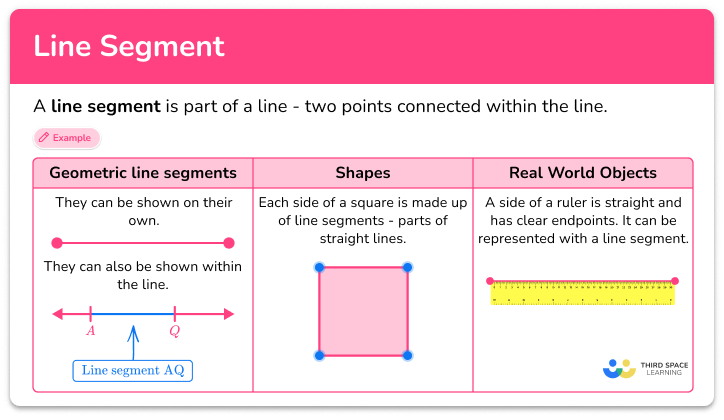Line segment

Line segment step-by-step topic guide, detailed examples, practice questions, teaching tips and FAQs.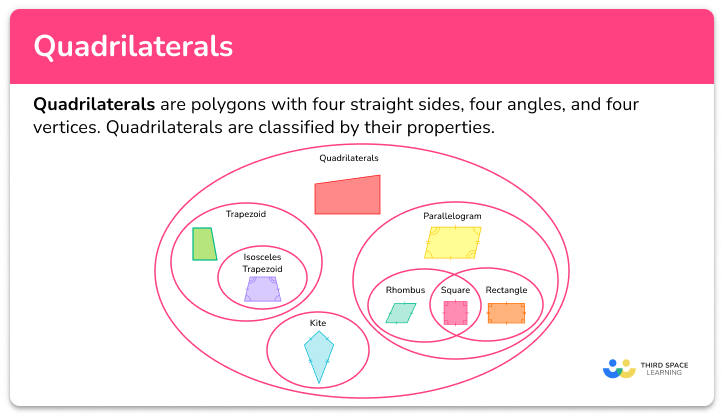Quadrilateral step-by-step topic guide, detailed examples, practice questions, teaching tips and FAQs.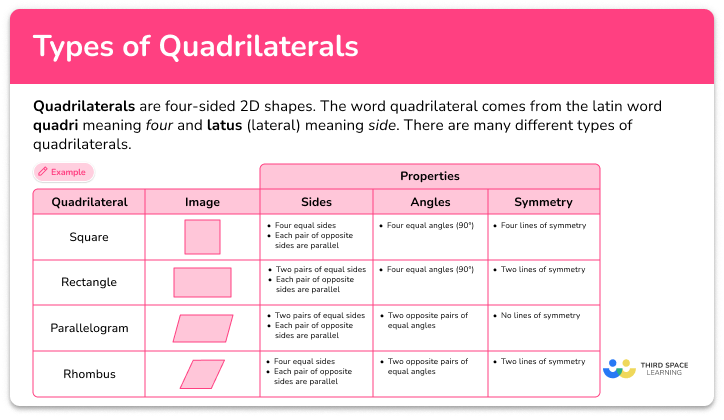Types of quadrilaterals step-by-step topic guide, detailed examples, practice questions, teaching tips and FAQs.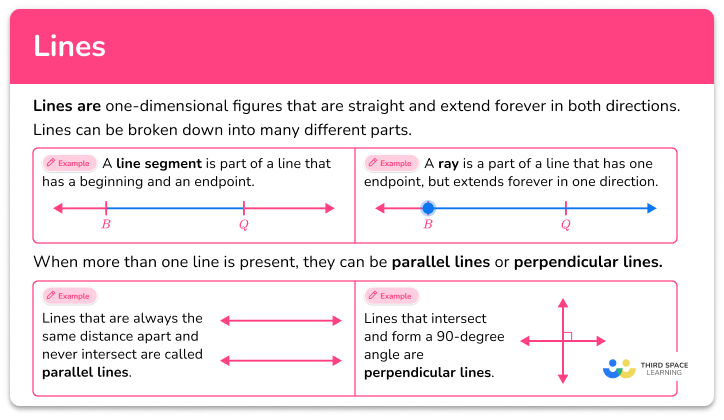Lines

Lines step-by-step topic guide, detailed examples, practice questions, teaching tips and FAQs.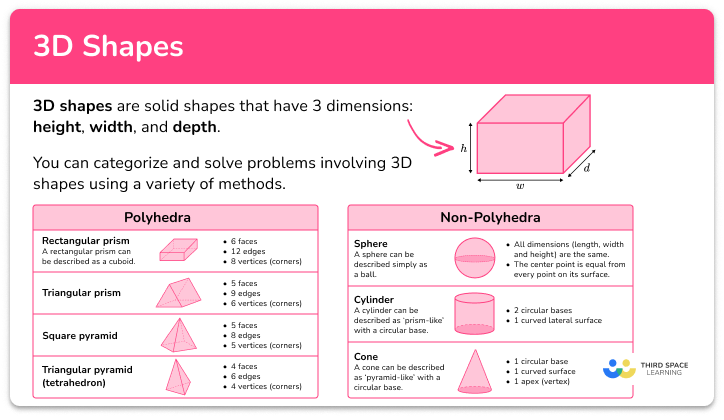3D shapes

3D shapes step-by-step topic guide, detailed examples, practice questions, teaching tips and FAQs.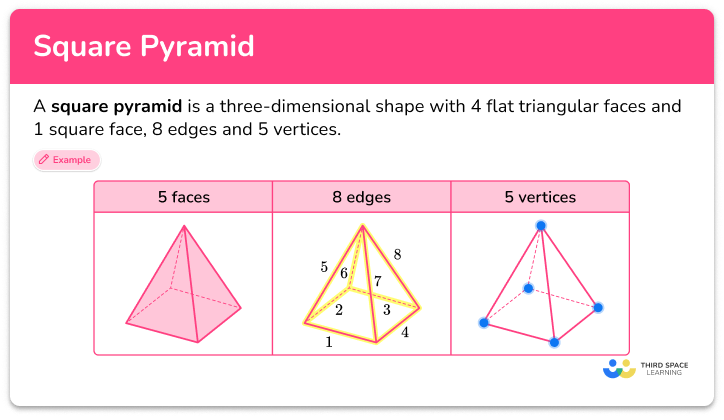Square pyramid

Square pyramid step-by-step topic guide, detailed examples, practice questions, teaching tips and FAQs.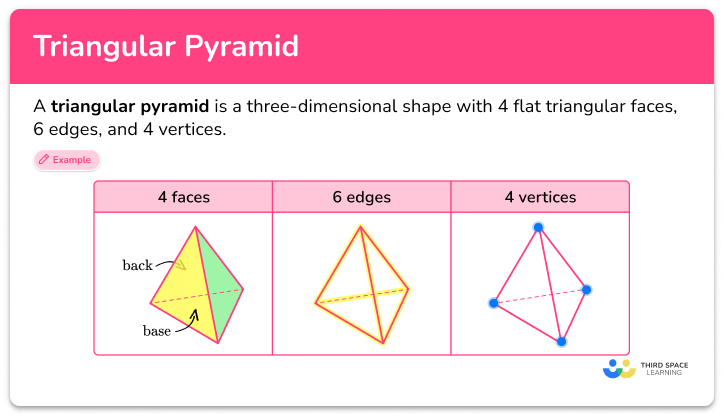Triangular pyramid

Triangular pyramid step-by-step topic guide, detailed examples, practice questions, teaching tips and FAQs.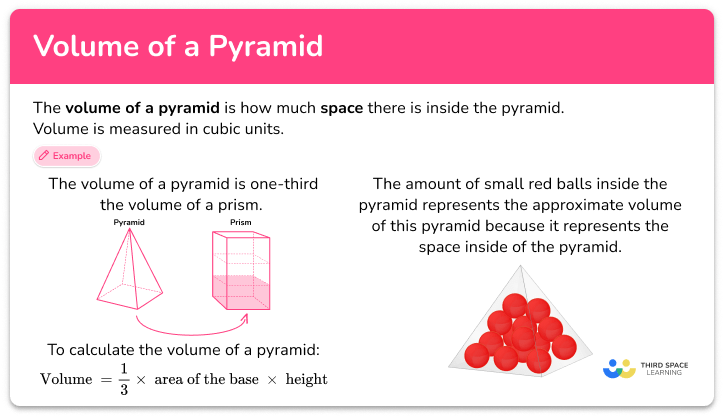Volume of a pyramid

Volume of a pyramid step-by-step topic guide, detailed examples, practice questions, teaching tips and FAQs.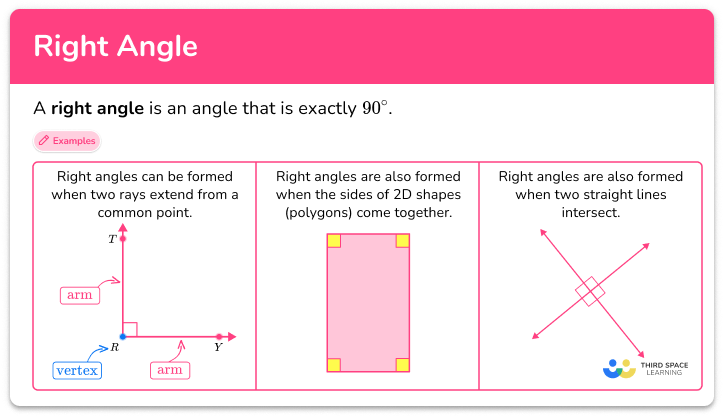Right angle

Right angle step-by-step topic guide, detailed examples, practice questions, teaching tips and FAQs.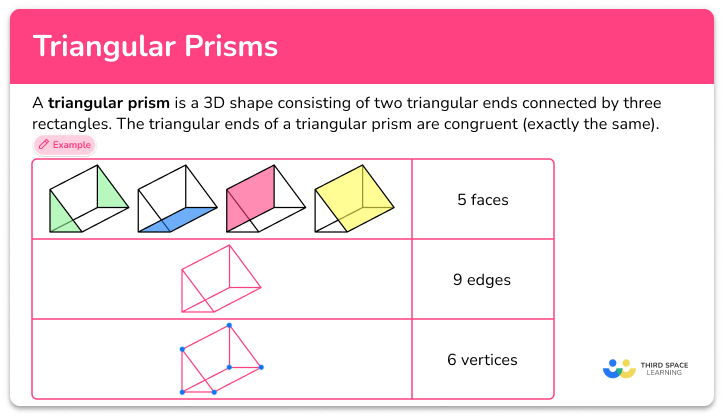Triangular prism

Triangular prism step-by-step topic guide, detailed examples, practice questions, teaching tips and FAQs.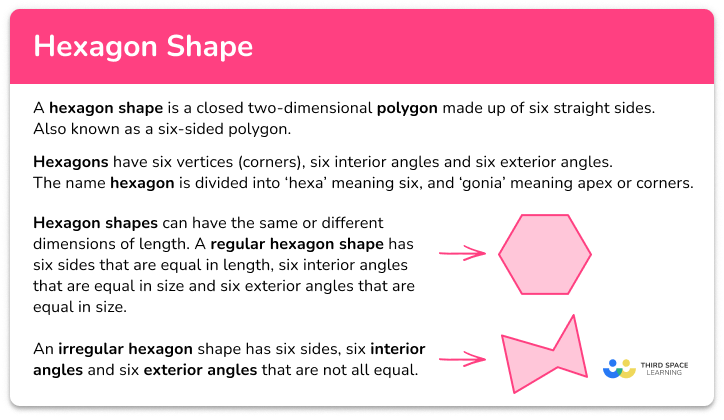Hexagon shape

Hexagon shape step-by-step topic guide, detailed examples, practice questions, teaching tips and FAQs.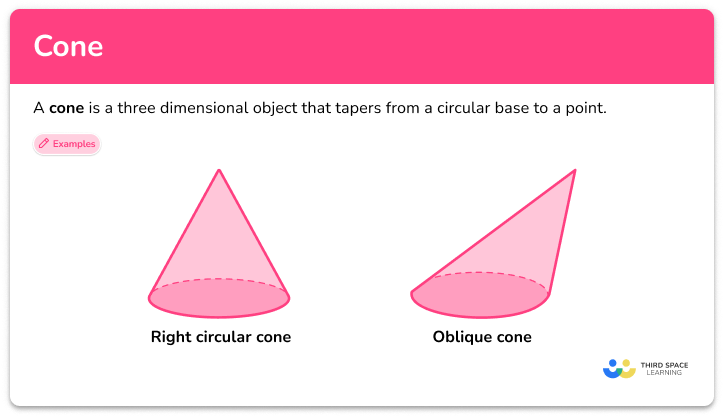Cone

Cone step-by-step topic guide, detailed examples, practice questions, teaching tips and FAQs.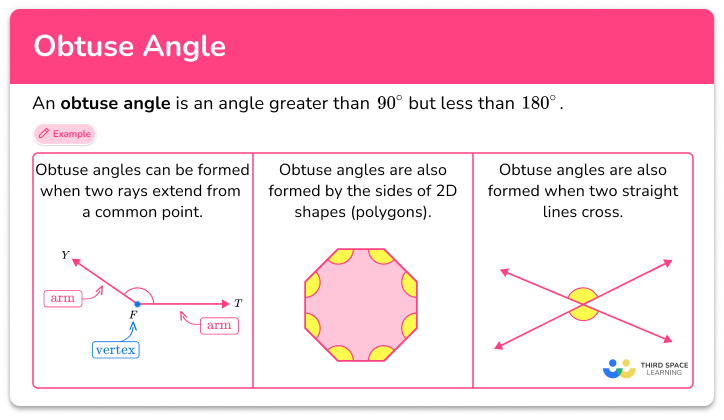Obtuse angle

Obtuse angle step-by-step topic guide, detailed examples, practice questions, teaching tips and FAQs.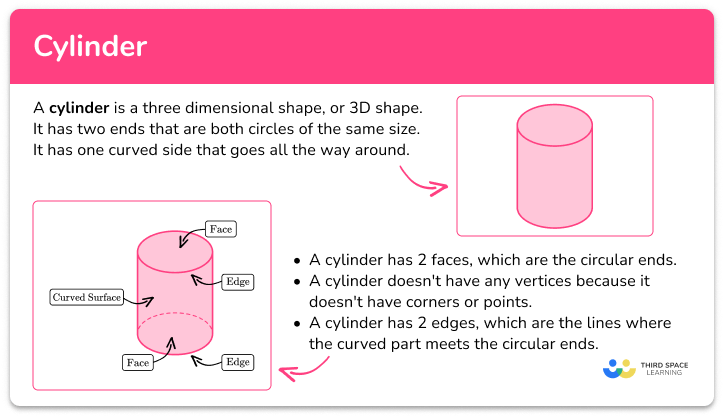Cylinder

Cylinder step-by-step topic guide, detailed examples, practice questions, teaching tips and FAQs.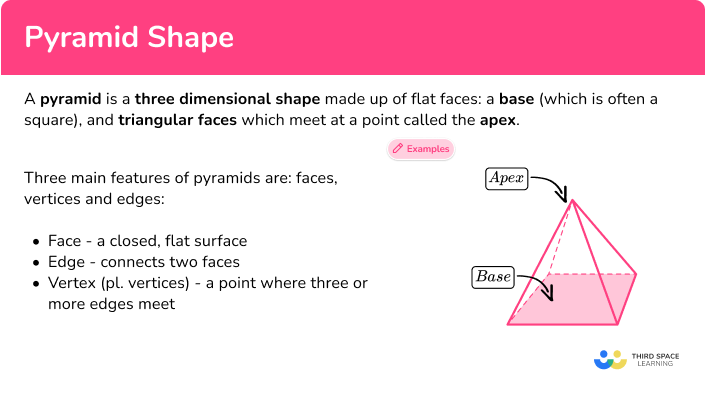Pyramid shape

Pyramid shape step-by-step topic guide, detailed examples, practice questions, teaching tips and FAQs.